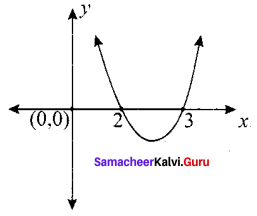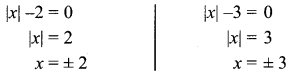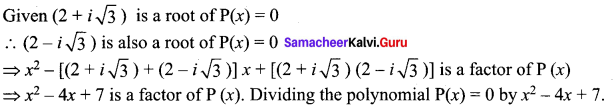# Samacheer Kalvi 12th Maths Solutions Chapter 3 Theory of Equations Ex 3.6

## Tamilnadu Samacheer Kalvi 12th Maths Solutions Chapter 3 Theory of Equations Ex 3.6

Question 1.
Discuss the maximum possible number of positive and negative roots of the polynomial equation 9x9 – 4x8 + 4x7 – 3x6 + 2x5 + x3 + 7x2 + 7x + 2 = 0.
Solution:
P(x) = 9x9 – 4x8 + 4x7 – 3x6 + 2x5 + x3 + 7x2 + 7x + 2
The number of sign changes in P(x) is 4.
∴P(x) has atmost 4 positive roots.
P(-x) = -9x9 – 4x8 – 4x7 – 3x6 – 2x5 – x3 + 7x2 – 7x + 2
The number of sign changes in P(-x) is 3.
P(x) has almost 3 negative roots. Since the difference between the number of sign changes in co-efficient P(-x) and the number of negative roots of the polynomial P(x) is even.
The number of negative roots = at most 2.

Question 2.
Discuss the maximum possible number of positive and negative zeros of the polynomials x2 – 5x + 6 and x2 – 5x + 16. Also, draw a rough sketch of the graphs.
Solution:
P(x) = x2 – 5x + 6
The number of sign changes in P(x) is 2.
P(x) has atmost 2 positive roots. P(-x) = x2 + 5x + 6.
The number of sign changes in P(-x) is 0.
∴ P (x) has no negative roots. P (x) = x2 – 5x + 16Question 3.
Show that the equation x9 – 5x5 + 4x4 + 2x2 +1 = 0 has atleast 6 imaginary solutions.
Solution:
P(x) = x9 – 5x5 + 4x4 + 2x2 + 1
(i) The number of sign changes in P(x) is 2. The number of positive roots is atmost 2.
(ii) P(-x) = -x9 + 5x5 + 4x4 + 2x2 + 1. The number of sign changes in P(-x) is 1. The number of negative roots of P (x) is atmost 1. Since the difference of number of sign changes in P(-x) and number of negative zeros is even.
P(x) has one negative root.
(iii) 0 is not the zero of the polynomial P(x). So the number of real roots is almost 3.
∴ The number of imaginary roots at least 6.

Question 4.
Determine the number of positive and negative roots of the equation x9 – 5x8 – 14x7 = 0.
Solution:
x9 – 5x8 – 14x7 = 0
P (x) = x9 – 5x8 – 14x7. The number of sign changes is P(x) is 1.
The number of positive roots is 1. P (-x) = -x9 – 5x8 + 14x7
The number of sign changes is P(-x) is one. The number of negative zero of P(-x) is 1. It is clear that 0 is a root of the equation.
∴ The number of the imaginary roots is at least 6.

Question 5.
Find the exact number of real zeros and imaginary of the polynomial x9 + 9x7 + 7x5 + 5x3 + 3x.
Solution:
P(x) = x9 + 9x7 + 7x5 + 5x3 + 3x.
There is no change in the sign of P(x) and P(-x), P(x) has no positive and no negative real roots, but 0 is the root of the polynomial equation P(x).

### Samacheer Kalvi 12th Maths Solutions Chapter 3 Theory of Equations Ex 3.6 Additional Problems

Question 1.
Find the maximum possible number of real roots of the equation, x5 – 6.x2 – 4x + 5 = 0.
Solution:
Let f(x) = x5 – 6x2 – 4x + 5
Check the terms when it changes sign
Number of changes = 2
∴ Maximum number of positive real roots = 2
f(-x) = (- x)5 – 6 (- x)2 – 4 (-x) + 5
= -x5 – 6x2 + 4x + 5
Check the terms when it changes signs.
Number of changes = 1
∴ Maximum number of negative real roots = 1
∴ Total maximum number of real roots = 2 + 1 = 3

Question 2.
Find the number of real roots of the equation, x2 + 5 |x| + 6 = 0.
Solution:
|x2| – 5|x| + 6 = 0
(|x| -2) (|x| -3) = 0It has four real roots. The real roots are 2, -2, 3, -3Question 3.
Find the real roots of the equation, x2 + 5 |x| + 6 = 0.
Solution:
x2 + 5 |x| + 6 = 0
Case (i) If x ≥ 0
x2 + 5x + 6 = 0
(x + 2) (x + 3) = 0
x = -2 and x = -3
Case (ii) If x < 0
x2 – 5x + 6 = 0
(x – 2) (x – 3) = 0
x = 2 and x = 3

Question 4.
Solve x4 – 4x2 + 8x + 35 = 0. Given (2 + $$i \sqrt{3}$$) is a root.
Solution:⇒ x2 – 4x + 7 is a factor of P (x). Dividing the polynomial P(x) = 0 by x2 – 4x + 7.
We get x2 + 4x + 5 = 0 is a other factor. The roots of x2 + 4x + 5 = 0 areQuestion 5.
Solve x4 – 5x3 + 4x2 + 8x – 8 = 0. Given (1 – $$\sqrt{5}$$) is a root of the polynomial equation.
Solution:
Since (1 – $$\sqrt{5}$$) is a root of the polynomial P(x) = 0
(1 + $$\sqrt{5}$$) is also a root of P (x) = 0
⇒ x2 – [(1 + $$\sqrt{5}$$) + (1 – $$\sqrt{5}$$)] x + (1 + $$\sqrt{5}$$ ) (1 – $$\sqrt{5}$$ ) = 0 is a factor of P(x) = 0 ⇒ x2 – 2x – 4 = 0 is a factor of P(x) = 0.
Dividing the polynomial by x2 – 2x – 4 = 0
We get the other factor x2 – 3x + 2 = 0
The roots of x2 – 3x + 2 = 0
(x – 2) (x – 1) = 0
x = 1, 2
The roots are 1, 2, 1 ± $$\sqrt{5}$$Question 6.
Find a polynomial equation of the lowest degree with rational co-efficient having $$\sqrt{3}$$, (1 – 2i) as two of its roots.
Solution:
When $$\sqrt{3}$$ is a root, – $$\sqrt{3}$$ will also be a root.
Now the quadratic equation with $$\sqrt{3}$$ , – $$\sqrt{3}$$ are roots is x2 – ($$\sqrt{3}$$ – $$\sqrt{3}$$)x + ($$\sqrt{3}$$)(-$$\sqrt{3}$$) = 0
(i.e) x2 – 3 = 0
When 1 – 2i is a root, 1 + 2i will be another root.
Now the quadratic equation with roots 1 – 2i and 1 + 2i is
x2 – (1 – 2i + 1 + 2i)x + (1 – 2i)(1 + 2i) = 0
(i.e) x2 – 2x + 5 = 0
∴ The equation with roots ± $$\sqrt{3}$$ and 1 ± 2i is (x2 – 3) (x2 – 2x + 5) = 0
(i.e) x4 – 2x3 + 5x2 – 3x2 + 6x – 15 = 0 ,
(i.e) x4 – 2x3 + 2x2 + 6x – 15 = 0